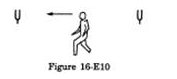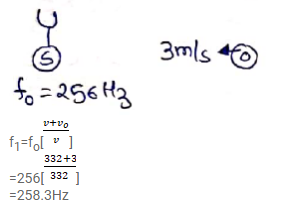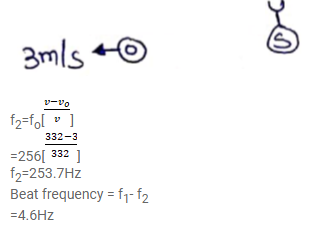# Two identical tuning forks vibrating at the same frequencyQuestion:

Two identical tuning forks vibrating at the same frequency $256 \mathrm{~Hz}$ are kept fixed at some distance apart. A listener runs between the forks at a speed of $3.0 \mathrm{~m} \mathrm{~s}^{-1}$ so that he approaches one tuning fork and recedes from the other figure. Find the beat frequency observed by the listener. Speed of sound in air $=332 \mathrm{~m} \mathrm{~s}^{-1}$.Solution: